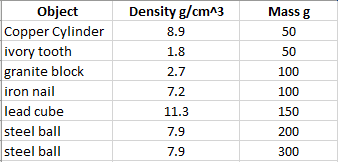# Is the buoyant force proportional to the mass?

1. The task is to figure out if the buoyant force is proportional to the mass of the object that is being sunk in the fluid (the fluid in this case water). I had an answer and that the buoyant force is proportional to the mass, but my answer only took into consideration objects with the same density.
I am trying to figure out if the buoyant force is still proportional to the mass even though the objects that are being sunk don't have the same density. I have tried different ways to solve it theoretically, but have yet to find a solution

## Homework Equations

F=pgV, archimedes principle.

## The Attempt at a Solution

I tried to solve the question with a graph. Having the mass on the x-axis and the buoyant force on the y-axis.
I still don't get any solutions and the graph doesn't really look right either.
The formula on y=(x/p)* P* g, P is the density of the fluid, p is the density of the object and x is the mass of the object.
I assumed the object would be dropped in water, so P=1, and g is of course 9.83. The equation became y=(x/a)*9.82. The graph showed that buoyant force was still proportional to the mass of the object, but I am extremely unsure if the way I did it was right or if it was the right answer.

TSny
Homework Helper
Gold Member
Object A: density 10 g/cm3 and total mass 100 g.
Object B: density 20 g/cm3 and total mass 200 g.

So, B has twice the mass of A.

If both are completely submerged in a fluid, how do their buoyant forces compare?

Well, It should be the same? because even if B has twice the mass of A, its density is also twice as that of A making the atoms more compact.

TSny
Homework Helper
Gold Member
Well, It should be the same? because even if B has twice the mass of A, its density is also twice as that of A making the atoms more compact.
Yes, the buoyant forces are the same even though the mass of one is twice the mass of the other.

What exactly does that prove? Sorry, I have a very slow mind.

TSny
Homework Helper
Gold Member
If y is proportional to x, then doubling x should double y. But you have an example where doubling the mass does not double the buoyant force.

TSny
Homework Helper
Gold Member
Here's a list of randomly chosen objects:If these objects are all submerged in water, find the buoyant force on each. Then graph the buoyant force vs mass. From your graph, would you say that the buoyant force is proportional to the mass of the object?

"The formula on y=(x/p)* P* g, P is the density of the fluid, p is the density of the object and x is the mass of the object.
I assumed the object would be dropped in water, so P=1, and g is of course 9.83. The equation became y=(x/a)*9.82."

The way this problem is setup is bit confusing. First off, density of water P = 1 g/cm3, and gravitational acceleration g = 9.8 m/s2. I would be consistent with units. You could use P = 1000 kg/m3. Second, I would use symbols that are bit easy to recognize. For example 'm' for mass and FB for buoyant force and so forth. This is not the big issue, however, since you are only trying to figure out how buoyant force is related to the mass. According to your expression, y=(x/p)* P* g, indeed y is proportional to x. But it is a false result! The reason is that you are keeping p, the density of the object, a constant.

You are attempting to change the mass x, keeping the volume constant, without changing the density! This is not possible. In other words, x and p are inter-related and that's why you are getting a false result. If you look at TSny's answer, he/she is also indirectly stating this by choosing materials of different densities!

By the way I like this problem for an inquiry type lab!

Cindy

Chestermiller
Mentor
F=pgV, archimedes principle.
In this formula for the buoyant force, ##\rho## is (a) the density of the object or (b) the density of the fluid that the object is submerged in? In this formula, V represents (a) the volume of the object or (b) the volume of fluid that has been displaced?

Yes

haruspex